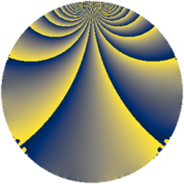# Properties

 Label 3724.1.bbLevel $3724$ Weight $1$ Character orbit 3724.bb Rep. character $\chi_{3724}(2059,\cdot)$ Character field $\Q(\zeta_{6})$ Dimension $8$ Newform subspaces $2$ Sturm bound $560$ Trace bound $6$

# Related objects

## Defining parameters

 Level: $$N$$ $$=$$ $$3724 = 2^{2} \cdot 7^{2} \cdot 19$$ Weight: $$k$$ $$=$$ $$1$$ Character orbit: $$[\chi]$$ $$=$$ 3724.bb (of order $$6$$ and degree $$2$$) Character conductor: $$\operatorname{cond}(\chi)$$ $$=$$ $$76$$ Character field: $$\Q(\zeta_{6})$$ Newform subspaces: $$2$$ Sturm bound: $$560$$ Trace bound: $$6$$

## Dimensions

The following table gives the dimensions of various subspaces of $$M_{1}(3724, [\chi])$$.

Total New Old
Modular forms 40 28 12
Cusp forms 8 8 0
Eisenstein series 32 20 12

The following table gives the dimensions of subspaces with specified projective image type.

$$D_n$$ $$A_4$$ $$S_4$$ $$A_5$$
Dimension 0 8 0 0

## Trace form

 $$8q + 4q^{4} + O(q^{10})$$ $$8q + 4q^{4} - 4q^{16} - 4q^{22} + 4q^{25} - 4q^{29} + 8q^{37} - 8q^{46} - 4q^{57} - 8q^{64} - 4q^{78} + 4q^{81} + 4q^{86} - 8q^{88} + 4q^{93} + O(q^{100})$$

## Decomposition of $$S_{1}^{\mathrm{new}}(3724, [\chi])$$ into newform subspaces

Label Dim. $$A$$ Field Image CM RM Traces $q$-expansion
$$a_2$$ $$a_3$$ $$a_5$$ $$a_7$$
3724.1.bb.a $$4$$ $$1.859$$ $$\Q(\zeta_{12})$$ $$A_{4}$$ None None $$0$$ $$0$$ $$0$$ $$0$$ $$q-\zeta_{12}^{5}q^{2}+\zeta_{12}^{5}q^{3}-\zeta_{12}^{4}q^{4}+\cdots$$
3724.1.bb.b $$4$$ $$1.859$$ $$\Q(\zeta_{12})$$ $$A_{4}$$ None None $$0$$ $$0$$ $$0$$ $$0$$ $$q+\zeta_{12}^{5}q^{2}+\zeta_{12}^{5}q^{3}-\zeta_{12}^{4}q^{4}+\cdots$$